## RD Sharma Class 10 Solutions Chapter 6 Co-ordinate Geometry Ex 6.5

These Solutions are part of RD Sharma Class 10 Solutions. Here we have given RD Sharma Class 10 Solutions Chapter 6 Co-ordinate Geometry Ex 6.5

Other Exercises

Question 1.
Find the area of a triangle whose vertices are :
(i) (6, 3), (-3, 5) and (4, -2)
(ii) ($${ at }_{ 1 }^{ 2 }$$, 2at1), ($${ at }_{ 2 }^{ 2 }$$, 2at2) and ($${ at }_{ 3 }^{ 2 }$$, 2at3)
(iii) (a, c + a), (a, c) and (-a, c – a)
Solution:
(i) Co-ordinates of ∆ABC are A (6, 3), B (-3, 5) and C (4, -2)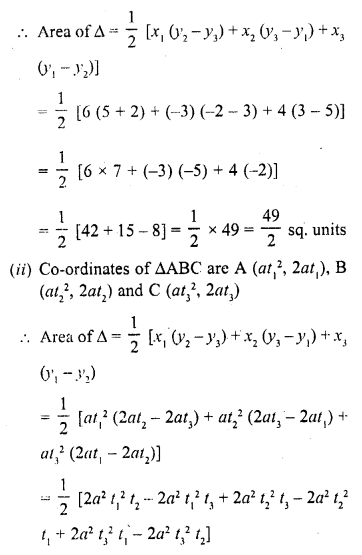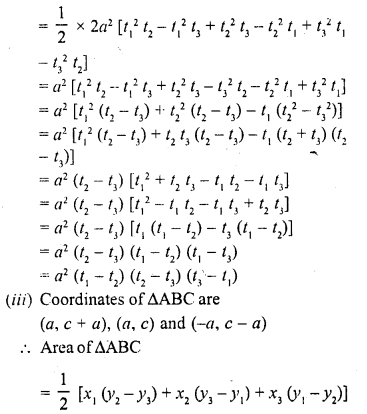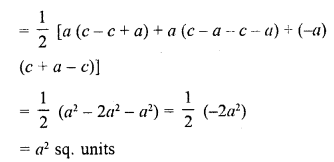Question 2.
Find the area of the quadrilaterals, the coordinates of whose vertices are
(i) (-3, 2), (5, 4), (7, -6) and (-5, -4)
(ii) (1, 2), (6, 2), (5, 3) and (3, 4)
(iii) (-4, -2), (-3, -5), (3, -2), (2, 3) (C.B.S.E. 2009)
Solution:
(i) Let vertices of quadrilateral ABCD be A (-3, 2), B (5, 4), C (7, -6) and D (-5, -4)
Join AC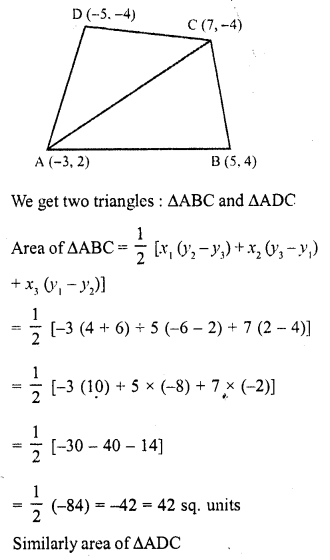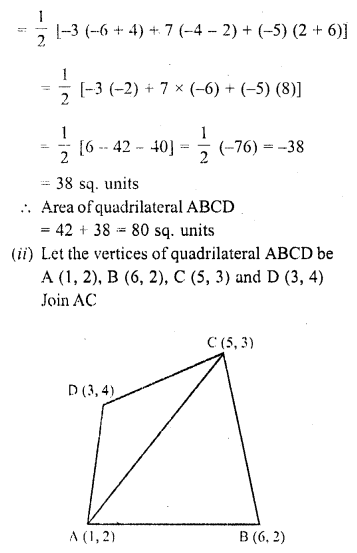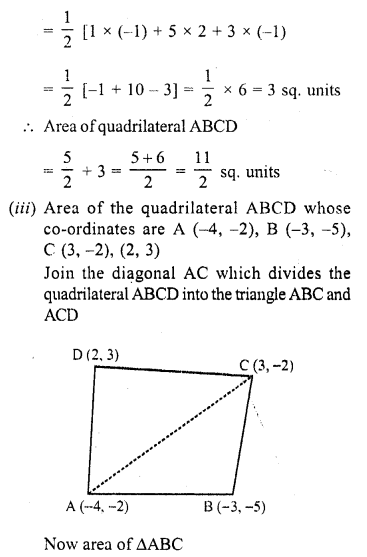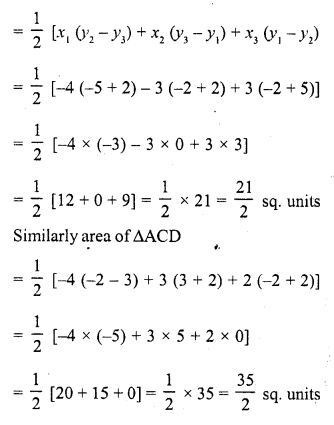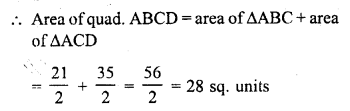Question 3.
The four vertices of a quadrilaterals are (1, 2), (-5, 6), (7, -4) and (k, -2) taken in order. If the area of the quadrilateral is zero, find the value of k ?
Solution:
Let the vertices of quadrilateral ABCD be
A (1, 2), B (-5, 6), C (7, -4) and D (k, -2)
Join AC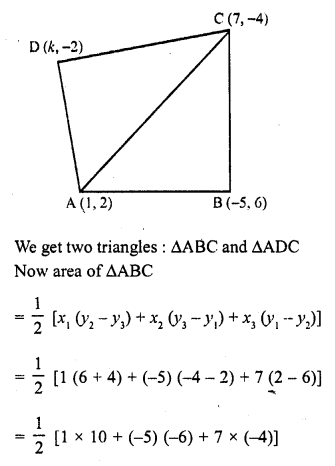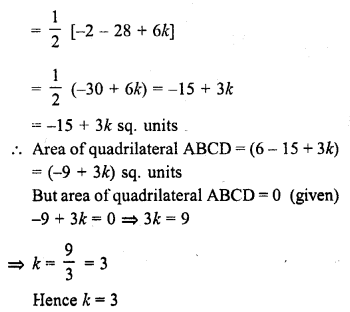Question 4.
The vertices of ∆ABC are (-2, 1), (5, 4) and (2, -3) respectively. Find the area of the triangle and the length of the altitude through A.
Solution:
Vertices of ∆ABC are A (-2, 1), B (5, 4) and C (2, -3) and AD ⊥ BC, let AD = h
Now area of ∆ABCQuestion 5.
Show that the following sets of points are collinear
(a) (2, 5), (4, 6) and (8, 8)
(b) (1, -1), (2, 1) and (4, 5)
Solution:
We know that points are collinear if the area of the triangle formed by them is zero
(a) Vertices of ∆ABC are (2, 5), (4, 6) and (8, 8)Question 6.
Find the area of a quadrilateral ABCD, the coordinates of whose varities are A (-3, 2), B (5, 4), C (7, -6) and D (-5, -4). [CBSE 2016]
Solution:
= area of ∆ABC + area of ∆ACDQuestion 7.
In ∆ABC, the coordinates of vertex A are (0, -1) and D (1, 0) and E (0, 1) respectively the mid-points of the sides AB and AC. If F is the mid-point of side C, find the area of ∆DEF. [CBSE 2016]
Solution:
Let B (p, q), C (r, s) and F (x, y)
Mid-point of AB = Coordinates of D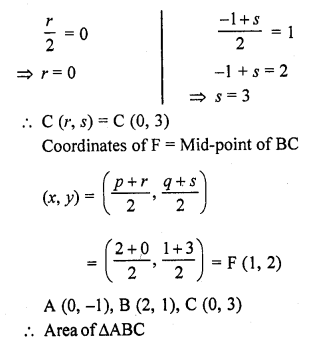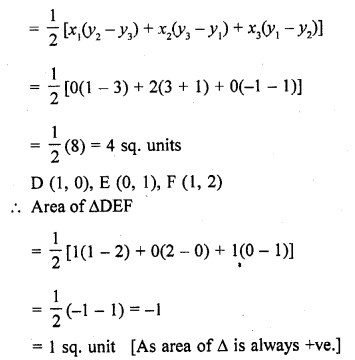Question 8.
Find the area of the triangle PQR with Q (3, 2) and the mid-points of the sides through Q being (2, -1) and (1, 2). [CBSE 2015]
Solution:
In ∆PQR, L and N are mid points of QR and QP respectively coordinates of Q are (3, 2) of L are (2, -1) and of N are (1, 2)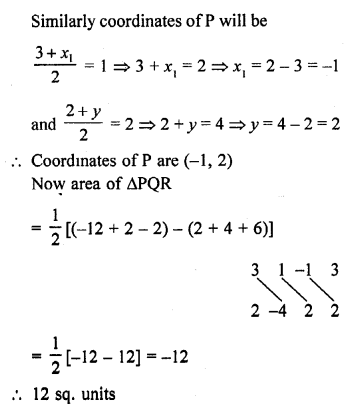Question 9.
If P (-5, -3), Q (-4, -6), R (2, -3) and S (1, 2) are the vertices of a quadrilateral PQRS, find its area. [CBSE 2015]
Solution:
P (-5, -3), Q (-4, -6), R (2, -3) and S (1,2) are the vertices of a quadrilateral PQRS
Join PR which forms two triangles PQR and PSRQuestion 10.
If A (-3, 5), B (-2, -7), C (1, -8) and D (6, 3) are the vertices of a quadrilateral ABCD, find its area. [CBSE 2014]
Solution:
A (-3, 5), B (-2, -7), C (1,-8) and D (6, 3) are the vertices of a quadrilateral ABCD
Join AC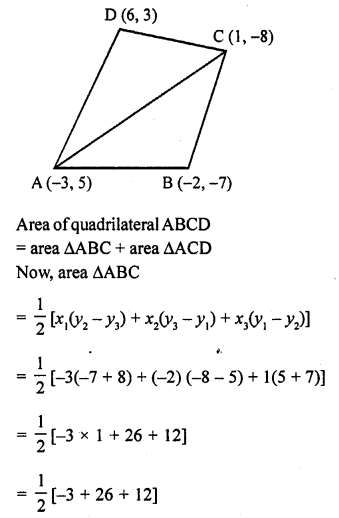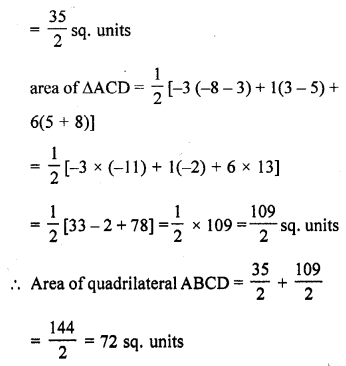Question 11.
For what value of ‘a’ the points (a, 1), (1, -1) and (11, 4) are collinear?
Solution:
Let the vertices of ∆ABC are A (a, 1), B (1, -1) and C (11, 4)Question 12.
Prove that the points (a, b), (a1, b1) and (a – a1, b – b1) are collinear if ab1 = a1b.
Solution: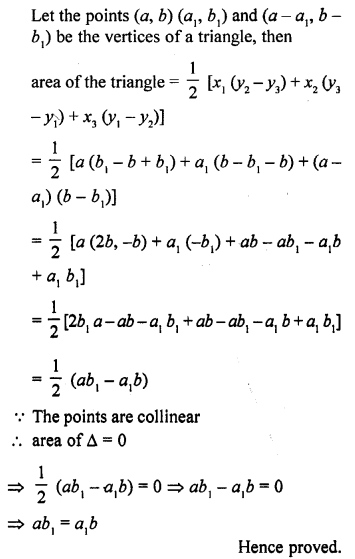Question 13.
If the vertices of a triangle are (1, -3), (4, p) and (-9, 7) and its area is 15 sq. units, find the value(s) of p. [CBSE 2012]
Solution:
The vertices of a triangle are (1, -3), (4, p) and (-9, 7) and area of triangle = 15 sq. units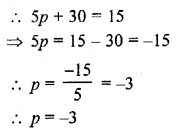Question 14.
If (x, y) be on the line joining the two points (1, -3) and (-4, 2), prove that x + y + 2 = 0.
Solution:
Point (x, y) be on the line joining the two points (1, -3) and (-4, 2)
Points (x, y), (1, -3) and (-4, 2) are collinear
Let the points (x, y) (1, -3) and (-4, 2) are the vertices of a triangle, then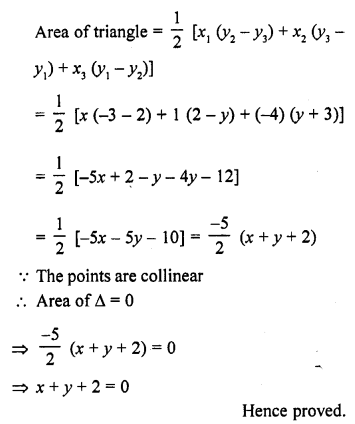Question 15.
Find the value of k if points (k, 3), (6, -2) and (-3, 4) are collinear. [CBSE 2008]
Solution:
Let the points (k, 3), (6, -2) and (-3, 4) be the vertices of a triangle, then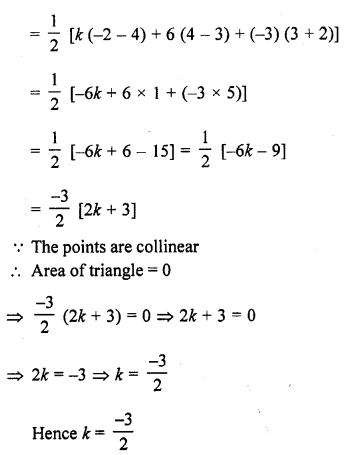Question 16.
Find the value of k, if the points A (7, -2), B (5, 1) and C (3, 2k) are collinear. [CBSE 2010]
Solution:
Points A (7, -2), B (5, 1) and C (3, 2k) are collinear
area of ∆ABC = 0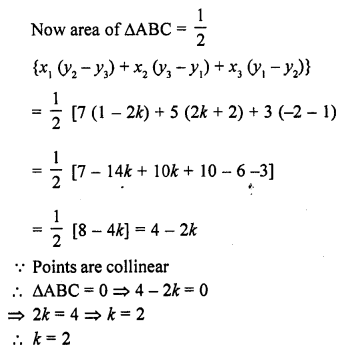Question 17.
If the point P (m, 3) lies on the line segment joining the points A ($$\frac { -2 }{ 5 }$$ , 6) and B (2, 8), find the value of m.
Solution: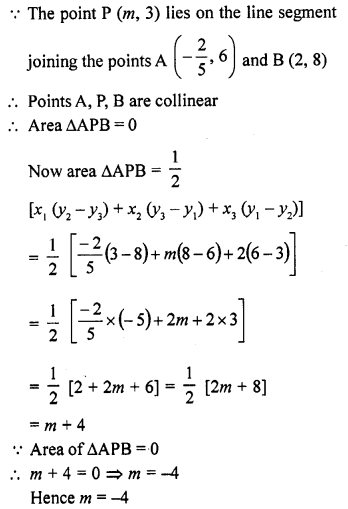Question 18.
If R (x, y) is a point on the line segment joining the points P (a, b) and Q (b, a), then prove that x + y = a + b. [CBSE 2010]
Solution:
Point R (x, y) lies on the line segment joining the points P (a, b) and Q (b, a)
Area of ∆PRQ = 0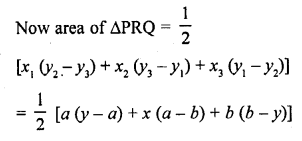Question 19.
Find the value of k, if the points A (8, 1), B (3, -4) and C (2, k) are collinear. [CBSE 2010]
Solution:
The points A (8, 1), B (3, -4) and C (2, k) are collinear
Area of ∆ABC = 0Question 20.
Find the value of a for which the area of the triangle formed by the points A (a, 2a), B (-2, 6) and C (3, 1) is 10 square units.
Solution: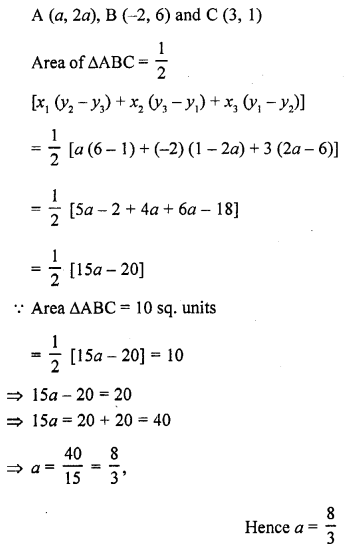Question 21.
If a ≠ b ≠ 0, prove that the points (a, a²), (b, b²), (0, 0) are never collinear. [CBSE 2017]
Solution:Question 22.
The area of a triangle is 5 sq. units. Two of its vertices are at (2, 1) and (3, -2). If the third vertex is ($$\frac { 7 }{ 2 }$$ , y), find y. [CBSE 2017]
Solution: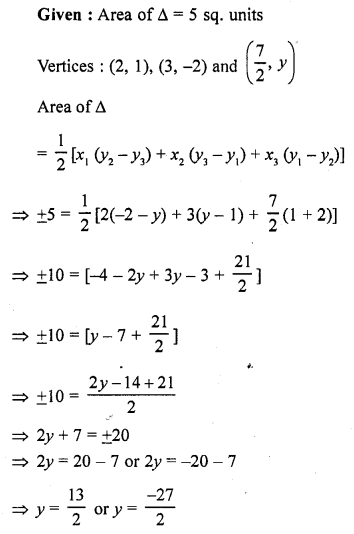Question 23.
Prove that the points (a, 0), (0, b) and (1, 1) are collinear if, $$\frac { 1 }{ a }$$ + $$\frac { 1 }{ b }$$ = 1.
Solution:
Let the points are A (a, 0), B (0, b) and C (1, 1) which form a triangleQuestion 24.
The point A divides the join of P (-5, 1) and Q (3, 5) in the ratio k : 1. Find the two values of k for which the area of ∆ABC where B is (1, 5) and C (7, -2) is equal to 2 units.
Solution:
Let the coordinates of A be (x, y) which divides the join of P (-5, 1) and Q (3, 5) in the ratio. Then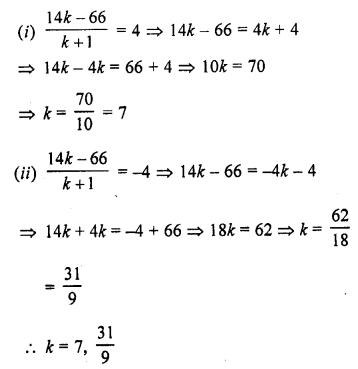Question 25.
The area of a triangle is 5. Two of its vertices are (2, 1) and (3, -2). The third vertex lies on y = x + 3. Find the third vertex.
Solution:
Let the coordinates of third vertex of the triangle be (x, y) and other two vertices are (2, 1) and (3, 2)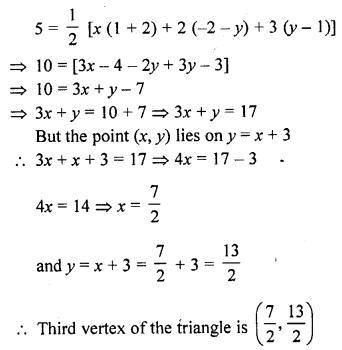Question 26.
If a ≠ b ≠ c, prove that the points (a, a²), (b, b²), (c, c²) can never be collinear.
Solution: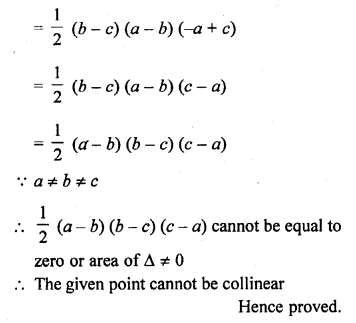Question 27.
Four points A (6, 3), B (-3, 5), C (4, -2) and D (x, 3x) are given in such a way that $$\frac { \triangle DBC }{ \triangle ABC } =\frac { 1 }{ 2 }$$ , find x?
Solution:
Let A (6, 3), B (-3, 5), C (4, -2) and D (x, 3x) are the vertices of quadrilateral ABCD
AC and BD are joined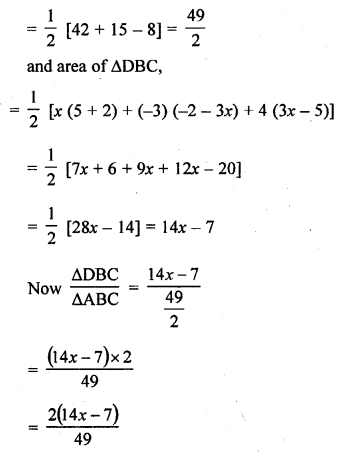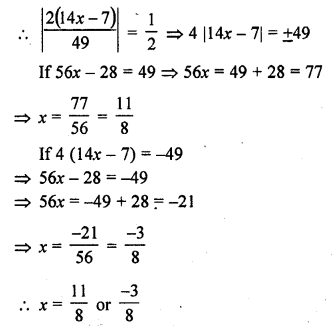Question 28.
If three points (x1, y1), (x2, y2), (x3, y3) lie on the same line, prove thatSolution:
Let the points (x1, y1), (x2, y2), (x3, y3) are the vertices of a triangleQuestion 29.
Find the area of a parallelogram ABCD if three of its vertices are A (2, 4), B (2 + √3, 5) and C (2, 6). [CBSE 2013]
Solution:
Three vertices of a ||gm ABCD are A (2, 4), B (2 + √3 , 5) and C (2, 6).
Draw one diagonal AC of ||gm ABCD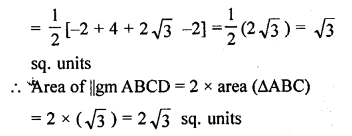Question 30.
Find the value (s) of k for which the points (3k – 1, k – 2), (k, k – 7) and (k – 1, -k – 2) are collinear. [CBSE 2014]
Solution: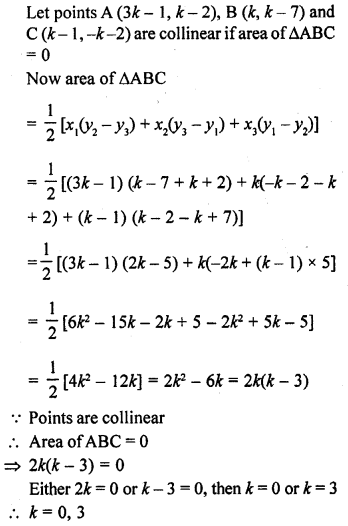Question 31.
If the points A (-1, -4), B (b, c) and C (5, -1) are collinear and 2b + c = 4, find the values of b and c. [CBSE 2014]
Solution:Question 32.
If the points A (-2, 1), B (a, b) and C (4, -1) are collinear and a – b = 1, find the values of a and 6. [CBSE 2014]
Solution:
Points A (-2, 1), B (a, b) and C (4, -1) are
collinear if area ∆ABC = 0
Now area of ∆ABC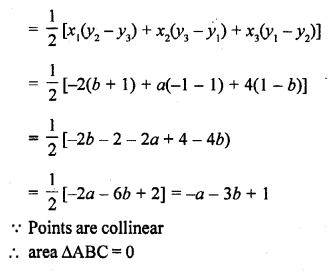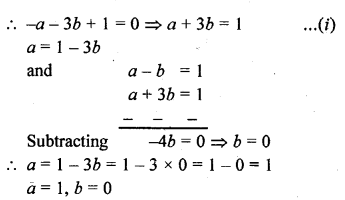Question 33.
If the points A (1, -2), B (2, 3), C (a, 2) and D (-4, -3) form a parallelogram, find the value of a and height of the parallelogram taking AB as base. [NCERT Exemplar]
Solution:
In parallelogram, we know that, diagonals bisects each other
i.e., mid-point of AC = mid-point of BD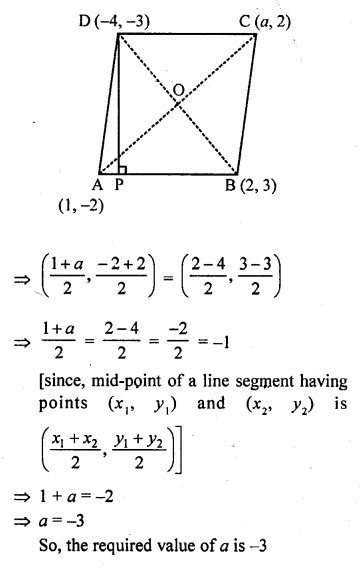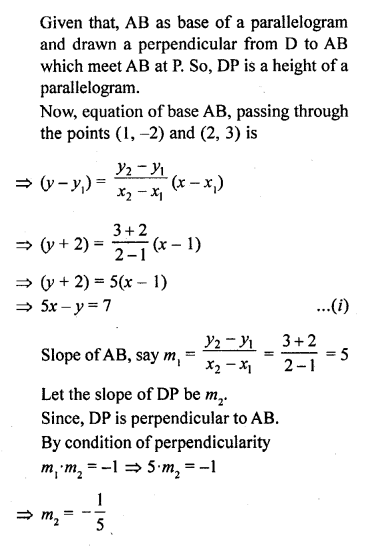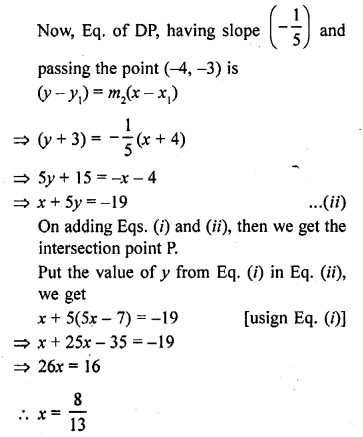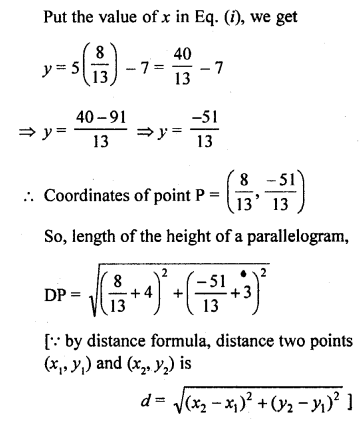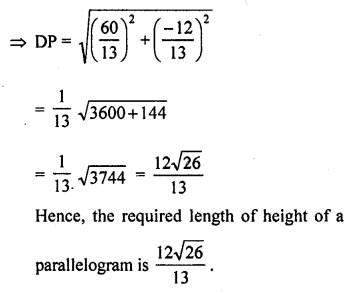Question 34.
A (6, 1), B (8, 2) and C (9, 4) are three vertices of a parallelogram ABCD. If E is the mid-point of DC, find the area of ∆ADE. [NCERT Exemplar]
Solution:
Given that, A (6,1), B (8,2) and C (9,4) are three vertices of a parallelogram ABCD.
Let the fourth vertex of parallelogram be (x, y).
We know that, the diagonal of a parallelogram bisect each other.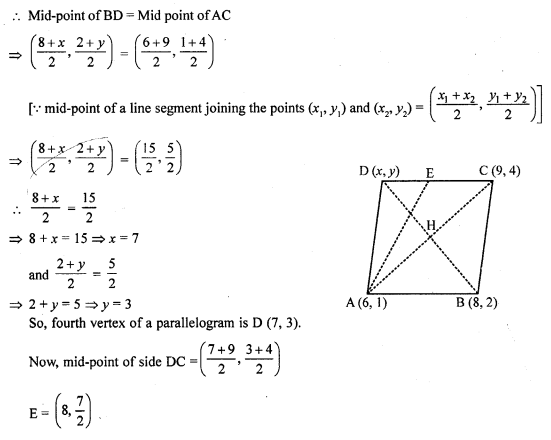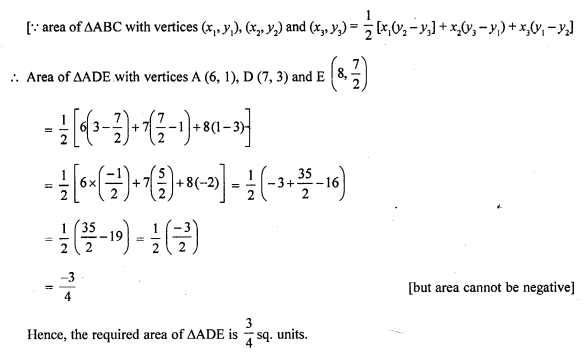Question 35.
If D ($$\frac { -1 }{ 2 }$$, $$\frac { 5 }{ 2 }$$) E (7, 3) and F ($$\frac { 7 }{ 2 }$$, $$\frac { 7 }{ 2 }$$) are the mid-points of sides of ∆ABC, find the area of ∆ABC. [NCERT Exemplar]
Solution: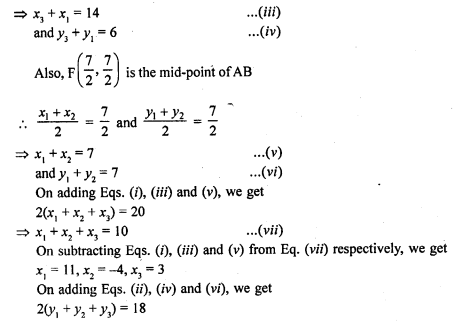Hope given RD Sharma Class 10 Solutions Chapter 6 Co-ordinate Geometry Ex 6.5 are helpful to complete your math homework.

If you have any doubts, please comment below. Learn Insta try to provide online math tutoring for you.